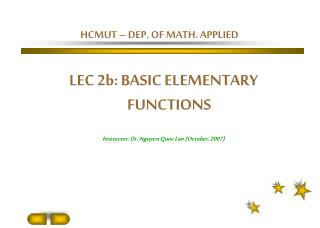DownloadDownload PresentationHCMUT – DEP. OF MATH. APPLIED

# HCMUT – DEP. OF MATH. APPLIED

Télécharger la présentation## HCMUT – DEP. OF MATH. APPLIED

- - - - - - - - - - - - - - - - - - - - - - - - - - - E N D - - - - - - - - - - - - - - - - - - - - - - - - - - -
##### Presentation Transcript

1. HCMUT – DEP. OF MATH. APPLIED LEC 2b: BASIC ELEMENTARY FUNCTIONS Instructor: Dr. Nguyen Quoc Lan (October, 2007)

2. CONTENT---------------------------------------------------------------------------------------------------------------------------------CONTENT--------------------------------------------------------------------------------------------------------------------------------- 1- POWER FUNCTION 8- HYPERBOLIC FUNCTION 2- ROOT FUNCTION 3- RATIONAL FUNCTION 4- TRIGONOMETRIC FUNCTION 5- EXPONENTIAL FUNCTION 6- LOGARITHMIC FUNCTION 7- INVERSE FUNCTION: TRIGONOMETRIC

3. Power Function The function y=xa , where a is a constant is called a power function (i) When a=n, a positive integer, the graph of f is similar to the parabola y=x2 if n is even and similar to the graph of y=x3 if n is odd However as n increases, the graph becomes flatter near 0 and steeper when x  1

4. The graphs of x2, x4, x6 on the left and those of x3, x5 on the right

5. Root functions (ii) a=1/n, where n is a positive integer Then is called a root function if n is even if n is odd The graph of f is similar to that of if n is even and similar to that of if n is odd

6. (1,1) (1,1)

7. (iii) When a=–1 , is the reciprocal function The graph is a hyperbola with the coordinate axes as its asymptotes

8. Rational functions A rational function is the ratio of two polynomials: Where P and Q are polynomials. The domain of f consists of all real number x such that Q(x)  0. is a rational function whose domain is {x/x 0}

9. Domain(f)={x/ x 2}

10. Trigonometric functions f(x)=sinx g(x)=cosx sinx and cosx are periodic functions with period 2 : sin(x + 2 ) = sinx, cos(x + 2 ) = cosx, for every x in R the domains of sinx and cosx are R, and their ranges are [-1,1]

11. Exponential functions These are functions of the form f(x)=ax, a > 0 y=2x y=(0.5)x

12. Logarithmic functions These are functions f(x)=logax, a > 0. They are inverse of exponential functions log2x log3x log10x log5x

13. Definition. A function f is a one-to-one function if: x1 x2  f(x1)  f(x2) 4 10 f is one-to-one 3 7 2 4 1 2 f g is not one-to-one : 2  3 but g(2) =g(3) 4 10 3 4 2 1 2 g

14. Example. Is the function f(x) = x3 one-to-one ? Solution1. If x13 = x23 then (x1 – x2)(x12+ x1x2+ x22) = 0  x1 = x2 because hencef(x) = x3 is one-to-one

15. Inverse functions Definition. Let f be a one-to-one function with domain A and range B. Then the inverse function f-1 has domain B and range A and is defined by: f -1(y) =x  f(x) = y, for all y in B domain( f–1) = range (f) range(f -1) = domain(f)

16. Example.Let f be the following function 4 -10 3 7 1 3 f A B

17. Then f-1 just reverses the effect of f 4 -10 3 7 1 3 f-1 A B

18. If we reverse to the independent variable x then: f -1(x) =y  f(y) = x, for all x in B f -1(f(x)) =x, for all x in A f(f -1(x)) =x, for all x in B How to find f –1 Step1 Write y = f(x) Step2 Solve this equation for x in terms of y Step3 Interchange x and y. The resulting equation is y = f -1(x)

19. Example.Find the inverse function of f(x) = x3 + 2 Solution. First write y = x3 + 2 Then solve this equation for x: Interchange x and y:

20. Application: Compute the integral Inverse trigonometric functions Question: When the trigonometric funtion y = sinx is one – to – one and how about its inverse function?

21. Application: Compute the integral Inverse trigonometric functions Considering analogicaly for the functions y = cosx, y = tgx, y = cotgx, we give the definition of three others inverse trigonometric functions

22. Hyperbolic functions The four next functions are called hyperbolic function We get directly hyperbolic formulas from all familiar trigonometric formulas by changing cosx to coshx and sinx to isinhx (i: imaginary number, i2 = –1)

23. Application: Compute the integral Hyperbolic formulas

24. Piecewise defined functions f(0)=1-0=1, f(1)=1-1=0 and f(2)=22=4 1 1 The graph consists of half a line with slope –1 and y-intercept 1; and part of the parabola y = x2 starting at the points (1,1) (excluded)